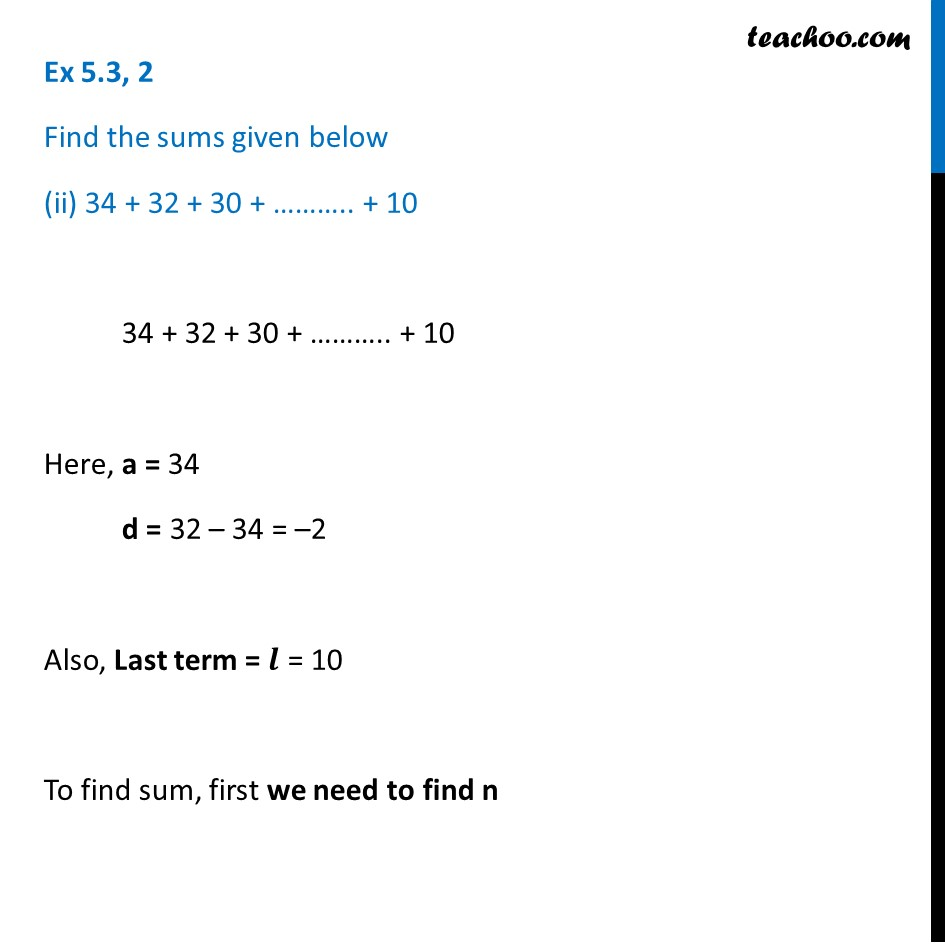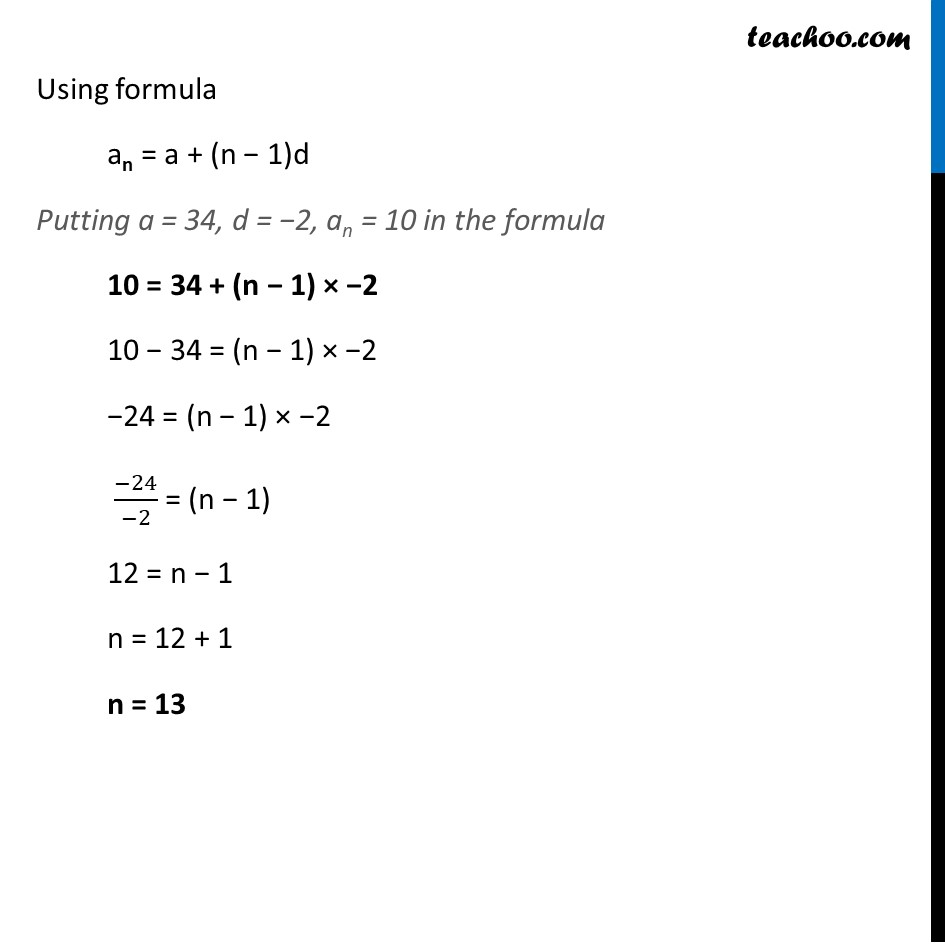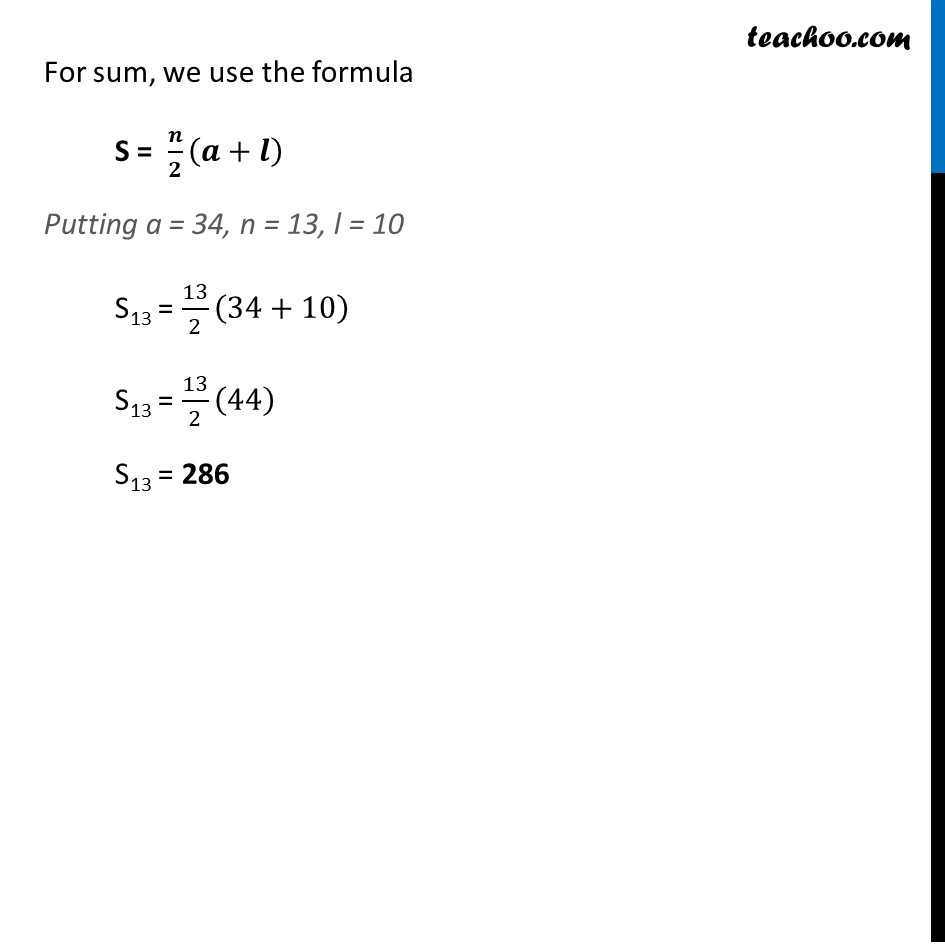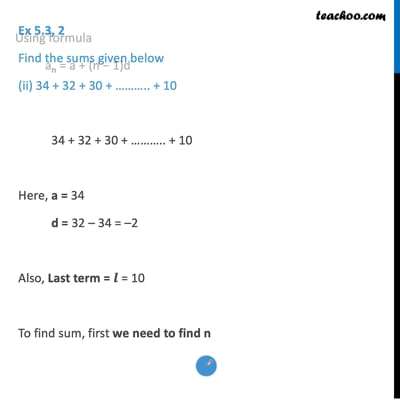Ex 5.3

Chapter 5 Class 10 Arithmetic Progressions (Term 2)
Serial order wiseThis video is only available for Teachoo black users

### Transcript

Ex 5.3, 2 Find the sums given below (ii) 34 + 32 + 30 + ……….. + 10 34 + 32 + 30 + ……….. + 10 Here, a = 34 d = 32 – 34 = –2 Also, Last term = 𝒍 = 10 To find sum, first we need to find n Using formula an = a + (n − 1)d Putting a = 34, d = −2, an = 10 in the formula 10 = 34 + (n − 1) × −2 10 − 34 = (n − 1) × −2 −24 = (n − 1) × −2 (−24)/(−2) = (n − 1) 12 = n − 1 n = 12 + 1 n = 13 For sum, we use the formula S = 𝒏/𝟐 (𝒂+𝒍) Putting a = 34, n = 13, l = 10 S13 = 13/2 (34+10) S13 = 13/2 (44) S13 = 286